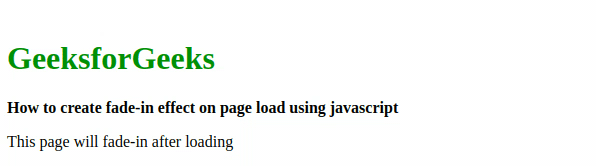Open in App
Not now

• Difficulty Level : Basic
• Last Updated : 30 Dec, 2022

The fade effect is described as the gradual increase and decrease in the opacity of the selected portion. In other words, the fade effect is termed as the increase and decrease in opacity with respect to time. When this effect is applied with a gradual increase in the opacity it is known as the fade-in effect. This is the effect that is used to apply fade-in on the selected part of the page with the selected interval of time.

We are using the setInterval() method and clearInterval() method in these logics.

Using setInterval() Method: On page loading, we are calling a function called the fadeIn() method. We are using the setInterval() method which takes two parameters one is function reference(which is the show() in this case) and the other is the timer(in number), which will call the show() function after every given time interval.

In the show() function, we are taking the property opacity in the variable name ‘opacity’ and increasing it by 0.1 every time the fadeIn() function is called. Initially, the opacity of the selected portion is set to 0. Two variables opacity and intervalID are declared inside the script tag. Variable opacity deals with the opacity of the project, while intervalID is used to call clearInterval() function

Then window.onload=fadeIn, is used to call the fadeIn() function automatically. The fadeIn() function calls an in-built method setInterval(), which takes two parameters, one function reference(show() in this case), and a time interval(to take reference of function after the selected interval). In setInterval(), the show() function is called after every 200 milliseconds in which we are checking the opacity of the body(variable body holds complete body with the help of id), if it is less than 1 there will be an increment of 0.1 in opacity otherwise clearInterval() function is called which will stop the function calling for show()

Note: The opacity of the selected portion is set to zero to understand the effect.

Example: This example shows the above-explained approach.

## JavaScript

 ` ` `    ``
` `    ``

GeeksforGeeks

` `    `` ` `        ``How to create fade-``in` `effect ` `        ``on page load using javascript ` `    `` ` `    ``

` `        ``This page will fade-``in` `after loading ` `    ``

` ` `  `    `` ` ``

Output:Using clearInterval() method: In this approach, complete logic is written inside a variable which is done with the help of the setInterval() method, here complete function is to be written in place of function reference. This approach is comparatively easy to understand. The default value of opacity is 1. So we have to set it to zero. to observe the output The window.onload=fadeIn is used to call the fadeIn() function automatically. Now declaring 3 variables in fadeIn() function:

• fade: It is to fetch the part on which the fade-in effect is to be applied.
• opacity: It is to deal with the opacity of fetched portion.
• intervalID: It is to deal with complete logic and terminate logic.

Now instead of writing a function reference, a complete function is to be written, which will be called over and over again after every 200 milliseconds to increase opacity. Now in the written function, the opacity of the fetched portion is compared with 1 on each call

• If opacity is found less than 1, the opacity will increase by 0.1 and be applied to the fetched portion.
• If opacity is found 1 or greater than 1, clearInterval() function is called to terminate function calls.

Example: This example shows the above-explained approach.

## JavaScript

 `<``body` `id``=``"body"` `style``=``"opacity:0;"``> ` `    ``<``br``> ` `    ``<``h1` `style``=``"color: green"``>GeeksforGeeks ` `    ``<``b``> ` `        ``How to create fade-in effect ` `        ``on page load using javascript ` `    `` ` `    ``<``p``> ` `        ``This page will fade-in after loading ` `    `` ` ` `  `    ``<``script` `type``=``"text/javascript"``> ` `        ``window.onload = fadeIn; ` `         `  `        ``function fadeIn() { ` `            ``var fade = document.getElementById("body"); ` `            ``var opacity = 0; ` `            ``var intervalID = setInterval(function() { ` `         `  `                ``if (opacity < ``1``) { ` `                    ``opacity` `= opacity + 0.1 ` `                    ``fade.style.opacity` `= opacity; ` `                ``} else { ` `                    ``clearInterval(intervalID); ` `                ``} ` `            ``}, 200); ` `        ``} ` `    `` ` ``

Output: This output video is in the loop, which is why it is not stopping after getting opacity 1. It loads again and again automatically.Note: If the increment value is greater than 0.1, it fade-in faster. If the time interval decreases then also it fade-in faster.

My Personal Notes arrow_drop_up
Related Articles